[TOC]

# 1. Regular Expressions

pb: pattern matching.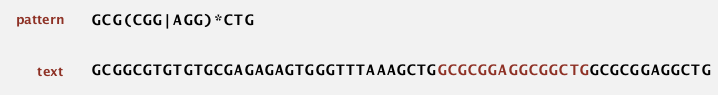### regular expression

Is a notation to specify a set of strings.
basic operations:

• concatenation
• or
• closure: "0 or more appearances of chars"
• parentheses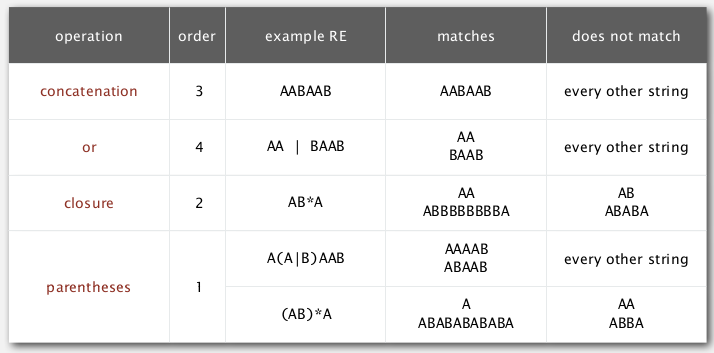additional operations (added for convinence):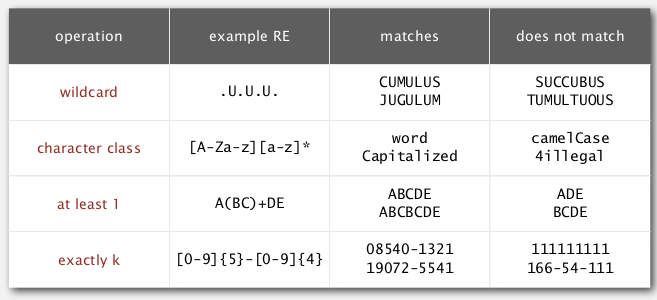ex. `[A-C]+` is equivalent to `(A|B|C)(A|B|C)*`.# 2. REs and NFAs

duality between RE and DFA:

• RE: to decribe a set of strings.
• DFA: machine to ecognize whether a string is in a given set.

[Kleene's therom]

For any DFA, there exists a RE that describes the same set of strings;
For any RE, there exists a DFA that recognizes the same set of strings.### first attempt of pattern matching

(Ken Tompson) same as KMP — no backup.
basic plan:

• construct the DFA
• simulate the DFA with text

bad news: DFA may have exponential nb of states.
⇒ change to NFA (nondeterministic finite automaton).

### NFA

• put RE into parentheses
• every char as a state (start=0, success=M) — 这里和之前的DFA很不一样: 之前是每个transition(edge)关联一个char, 这里是每个状态(node)关联一个char.
• epsilon-transition (red links below): change of machine state without scanning text
• match-transition (black links below): change state, but also have to scan next char in text, match transition is added after each alphabetic char
• success (accept) if any sequence of transitions (after scanning all text) end at state-M.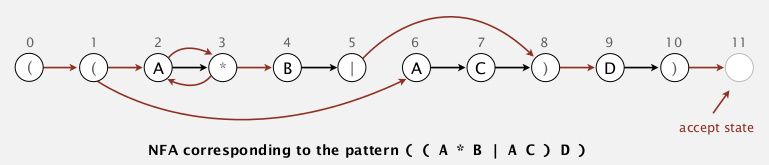example:
is "AAAABD" a match ?
→ yes. (和上一节substring的插图进行一下比对, 还是有很大不同)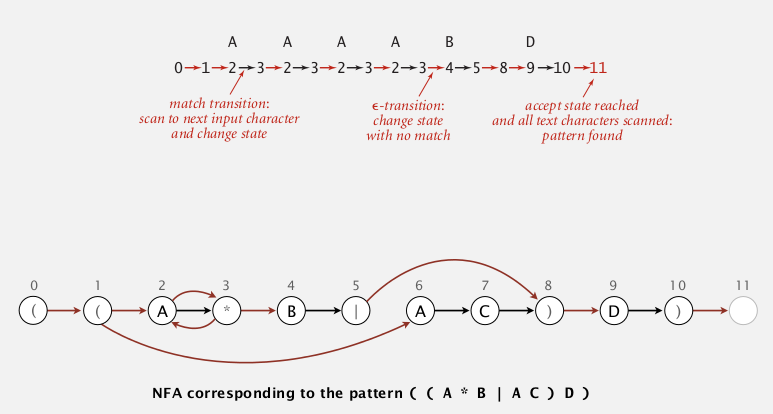pb: non-determinism
How to determine whether a string is a match of a NFA (ie. how to select the right sequence of transition) ?
⇒ sysematically consider all possible transition sequences.

# 3. NFA Simulation

• state names: 0 to M. (M+1 states in total, M=length of RE string).
• match-transitions: store in array `re[]` (the match transitions are naturally in order of the array).
• epsilon -transitions: store in a digraph `G`

idea:

maintain a set of all state that NFA could be in after reading first i chars in text.

at each iteration: check all reachable state wrt the transitions, then update reachable states.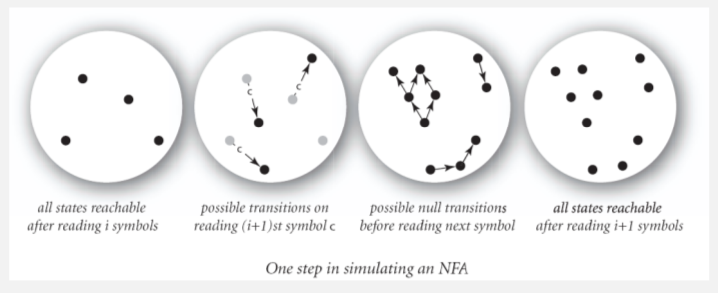### algorithm(for the NFA above, 注意为了方便已经加了 必要的括号)

[Algo]

• initial: `rs`(reachable state)=reachable state from state 0 (left parenthese) using epsilon trantisions
• consume a char in text:

• `nrs` (new-reachable-states) = empty set

• from all reachable state of this character: add next state using the match-transition to `nrs`
• add all reachable states (using epsilon transition) form the `nrs` set to `nrs`
• set `rs = nrs`, and consume the next char in text
• accept if at the end the state M is in `rs`

### concrete example

• init:• when matching A from text: state 2 or 6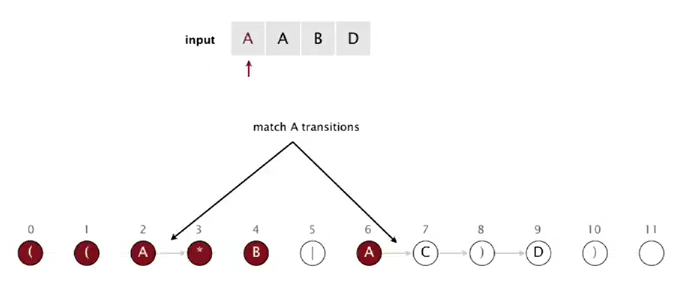using match transition of A, we can get to state 3 or 7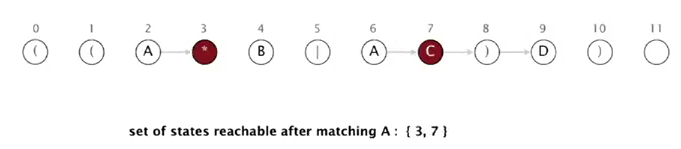if we add epsilon transitions:so reachable states after reading 1st A are: 2, 3, 4, 7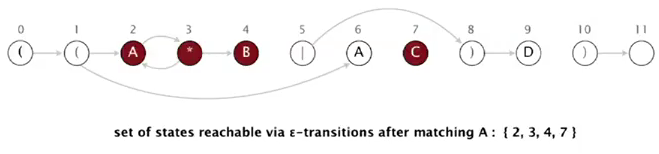• matching 2nd A from text: state 2

using match transition we can only get to state 3.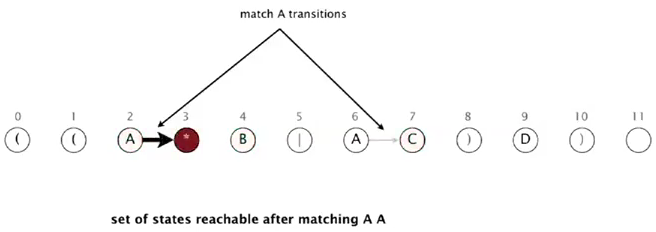using epsilon transitions from state 3: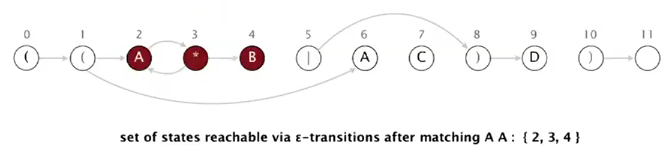(the only state after matching A is state 2 3 4)

• etc...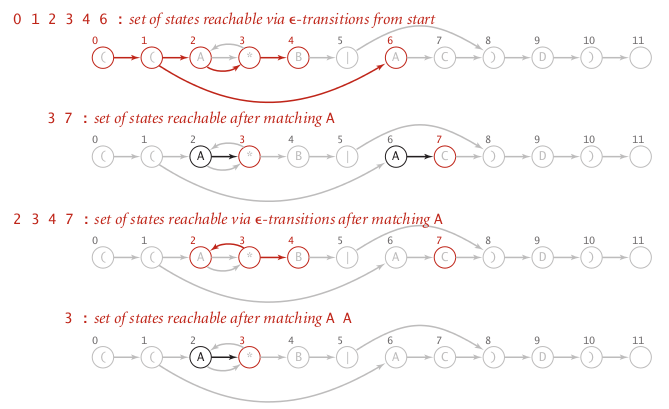### reachability

All reachable vertices from a set of source vertices → just DFS.
⇒ directly use the API from the digraph section: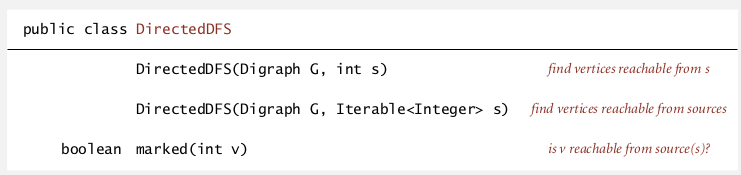running time linear to E+V

### Java implementation

API:

```public class NFA{
private int M;
private char[] re;
private Digraph G;// digraph of the epsilon-transitions
public NFA(String regexp){
M = regexp.length();
re = regexp.toCharArray();
G = buildEpsilonTransitionGraph();// helper function to build the graph G
}
public boolean matches(String text);// does text match the regexp?
private Digraph buildEpsilonTransitionGraph();// private helper function
}
```

The function `buildEpsilonTransitionGraph()`will be attacked in next session, for now we focus on the NFA simulation code — that is, the `mathes()` method.

For simplicity let's assume we have a function `reachableVertices(Digraph G, Bag<Integer> sourceSet)` and `reachableVertices(Digraph G, int source)` that gives the reachable states from (a set of) source vertices, including the sources. Or we can directly use the `DirectedDFS` api as listed above.

```    public boolean matches(String text){ //does text match the regexp?
Bag<Integer> rechableStates = reachableVertices(G,0);// init reachable
for(char c: text){
Bag<Integer> newRechableStatesBymatch = new Bag<Integer>();
for(int i:rechableStates)
if(re[i]==c || re[i]=='.')
newRechableStatesBymatch.add(i+1);// match transition
rechableStates = reachableVertices(G,newRechableStates);//epsilon transition
}
return reachableStates.contains(M);
}
```

(代码虽然短但是这个过程我理解了好久.. 另外上面的代码有点伪).

### Analysis

prop. the matches() method takes `O(MN)` time in worst case.

pf. N chars in text, each char can go through <= M states (DFS), and in the digraph, no node has >3 degree ⇒ number of edges <= 3M, so the time for each dfs is O(M), in total we have O(MN).

# 4. NFA Construction

→ construct the epsilon transition digraph.

### buiding a NFA from a re (parsing)

• states in a NFA: one state per char, plus an accept state (state M)
• alphabet state: chars in alphabet (`A, B, C, D`) → (implicitly) put a match transition to next state
• metacharacters: `( ) . * |` , 5 metacharacters in total

⇒ to deal with the metacharacters:

• paretheses `( )`

simply put a epsilon-transition to the next state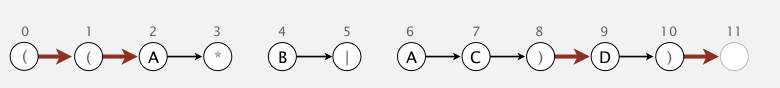• closure `*`

for each `*` state, add 3 transitions as below: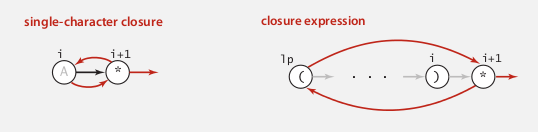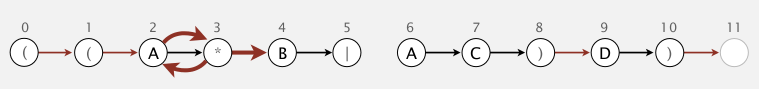• or `|`

or符号肯定在一个括号里面
add 2 epsilon transitions wrt parethese: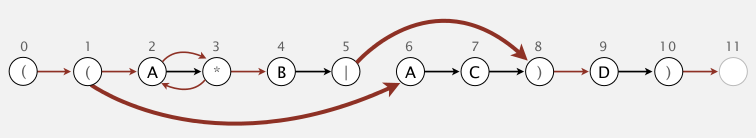### implementation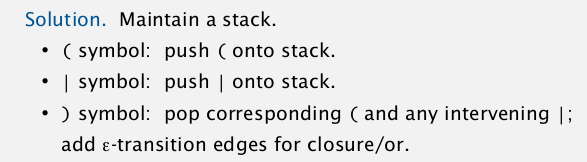• for alphabetic chars: do one-char lookahead → if next is `*`, add transitions.
• for left parenthese `(`: add transition to next state, and push to stack
• for or `|`: add transition to next state, and push to stack
• for right parenthese `)`: pop the stack to deal with or and lp; and also do lookahead.

code is not trival... look carefully:

```private Digraph buildEpsilonTransitionGraph(){// private helper function
Digraph G = new Digraph(M+1);
Stack<Integer> stk = new Stack<Integer>();
int lp;
for(int i=0; i<M; i++){
if(re[i]=='|' || re[i]=='(')
stk.push(i);
if(re[i]=='(' || re[i]==')' || re[i]=='*')
G.addEdge(i,i+1);
else if(re[i]==')'){// need to pop until get a lp
int j = stk.pop();
if(re[j]=='|'){
lp = stk.pop();
int or = j;
G.addEdge(lp, or+1);// add edge for the `or` case
G.addEdge(or, i);

}
else lp = j;
}
// do the lookahead:
if(re[i+1]=='*'){
if(re[i]==')'){ // case 1 of closure: a rp before `*`
G.addEdge(lp, i+1);
G.addEdge(i+1, lp);
}else{ // case 2 of closure: an alphabetic char before `*`
G.addEdge(i, i+1);
G.addEdge(i+1, i);
}
}
}// go through each char in re
return G;
}
```

### Analysis

prop. building an NFA takes linear time and space in M.
pf. for each char, the nb of operations is const.

# 5. Regular Expression Applications

### grep

"Generalized Regular Expression Print"
print out all lines (from stdin) having a substring of an RE.
⇒ equal to adding a `.*` to the beginning and end of the RE to make a match.

```public class GREP{
public void main(String[] args){
String re = ".*"+args+".*";
NFA nfa = new NFA(re);
while(StdIn.hasNextLine){
String line = StdIn.readLine();
if(nfa.matches(line)) StdOut.println(line);
}
}
}
```

the grep has NM worst case running time — same as brute force substring search — amazing...

grep application: crossword puzzles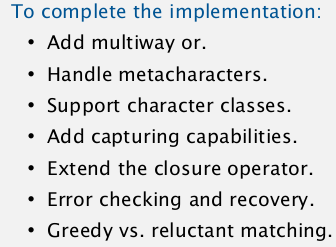### regexp in other languages

• unix: grep, awk
• script: python, perl• java: `String.matches(regexp)`### Harvesting information

goal: print all substrings of input that match an RE.
use `Pattern` and `Matcher` class in `java.util.regexp`.
first compile the regexp, then build the matcher
→ so that we can iterate through all matches of the input using `find()` and `group()` of the matcherCaveat: performance NOT guaranteed !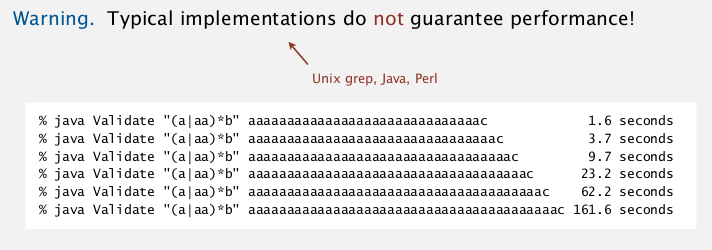→ exponential time growth!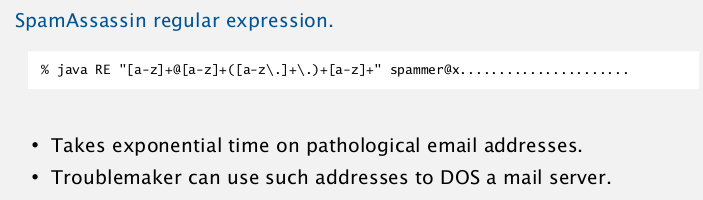### Not-so-regular expressions

"not rugular" means Kleene's Th doesn't hold
→ efficient performance not tractable......
back-reference
`\1` matches subexpressions that was matched earliser
limitations of regular languages: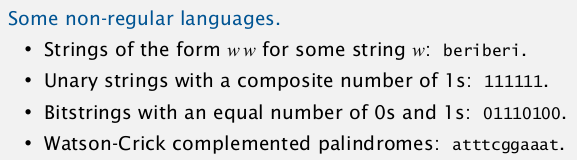### Summary

the substring and regexp are examples of compilers ! (from string to a NFA/DFA/bytecode)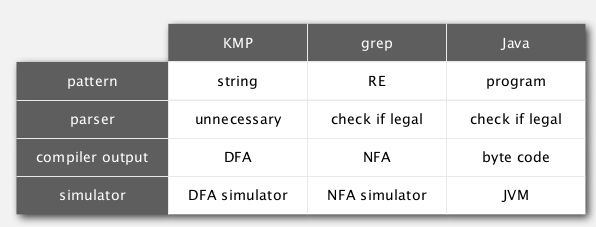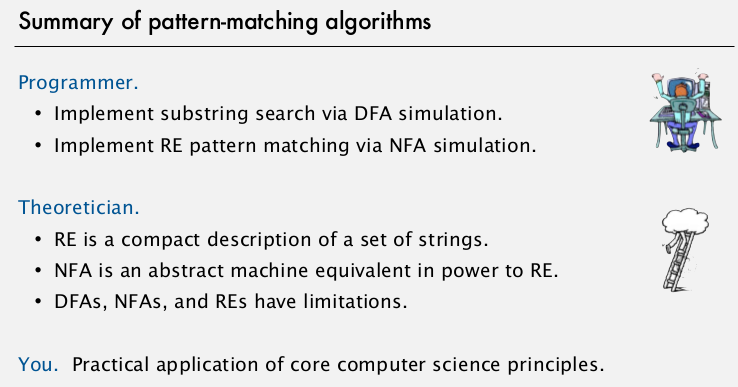#### Part 9 of series «Algorithms Princeton MOOC II»：

comments powered by Disqus